# canvas中的拖拽、缩放、旋转 (上) —— 数学知识准

jojo

3收藏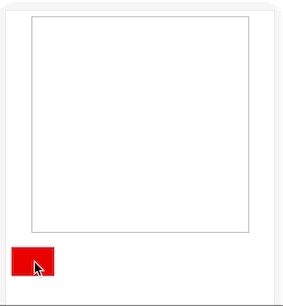canvas中的所有元素(图片、各种图形等)都是长方形的。这已经能覆盖大部分情况了。长方形四个顶点的坐标就能确定一个元素的位置了。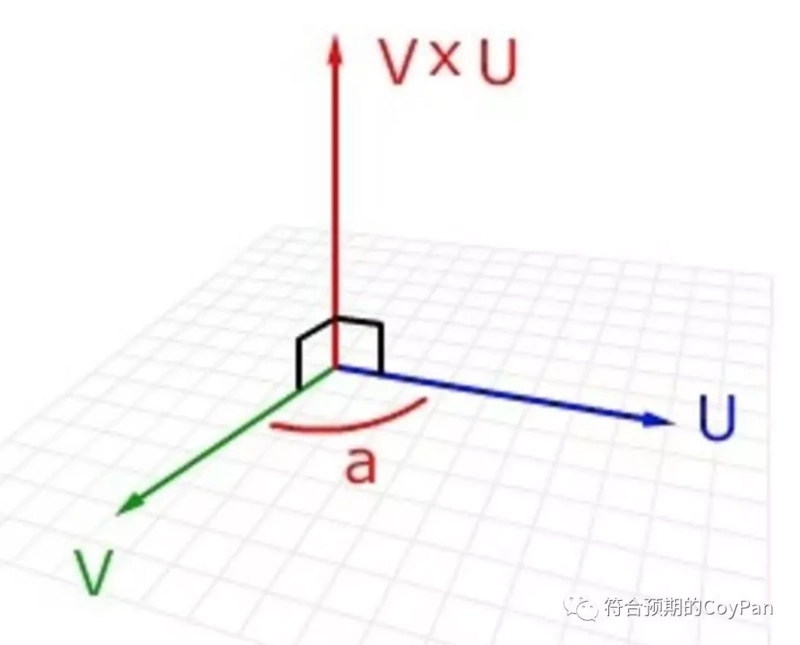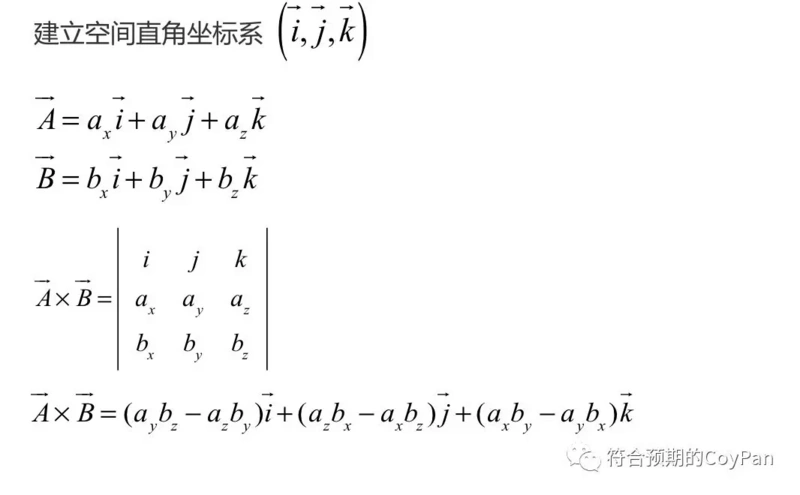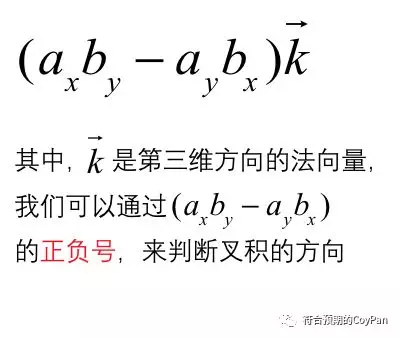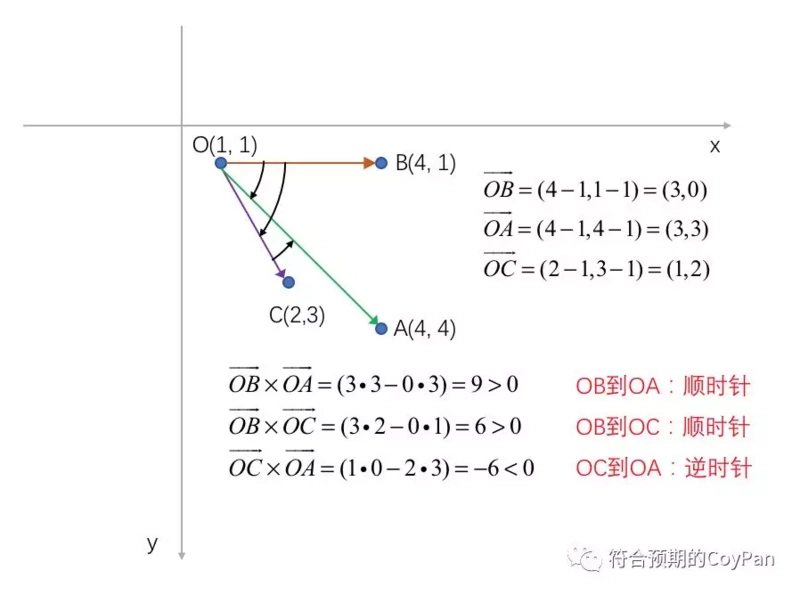``````/**
* 判断落点是否在长方形内
*
* @param {Array} point 落点坐标。 数组：[x, y]
* @param {Array} rect 长方形坐标, 按顺序分别是：左上、右上、左下、右下。
*                     数组：[[x1, y1], [x2, y2], [x3, y3], [x4, y4]]
*
* @return {boolean}
*/

function isPointInRect(point, rect) {
const [touchX, touchY] = point;
// 长方形四个点的坐标
const [[x1, y1], [x2, y2], [x3, y3], [x4, y4]] = rect;
// 四个向量
const v1 = [x1 - touchX, y1 - touchY];
const v2 = [x2 - touchX, y2 - touchY];
const v3 = [x3 - touchX, y3 - touchY];
const v4 = [x4 - touchX, y4 - touchY];
if(
(v1 * v2 - v2 * v1) > 0
&& (v2 * v4 -  v4 * v2) > 0
&& (v4 * v3 - v3 * v4) > 0
&& (v3 * v1 -  v1 * v3) > 0
){
return true;
}
return false;
}``````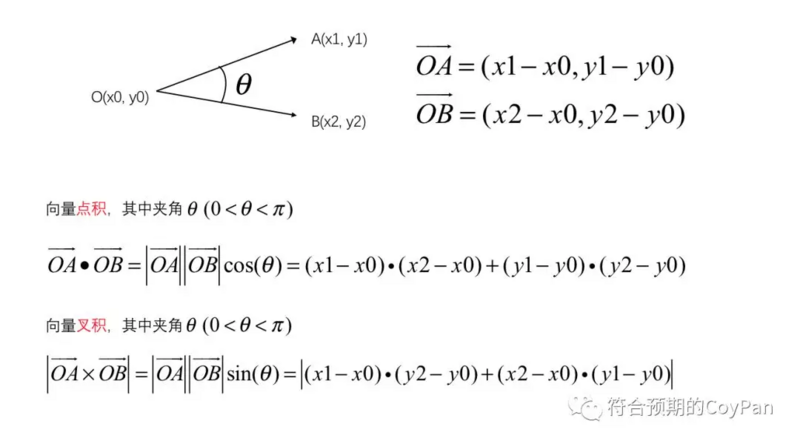1、一般以canvas中的元素的中心为旋转原点，用户在canvas中触摸移动时，通过事件监听函数得到的前后两次触摸点的位移是很小的，与旋转中心形成的向量夹角必然是小于90度的。

2、向量的叉积正负值可以确定旋转方向。

3、反正弦函数是在负90度到90度之间单调递增的。

``````/**
* 计算旋转角度
*
* @param {Array} centerPoint 旋转中心坐标
* @param {Array} startPoint 旋转起点
* @param {Array} endPoint 旋转终点
*
* @return {number} 旋转角度
*/

function getRotateAngle(centerPoint, startPoint, endPoint) {
const [centerX, centerY] = centerPoint;
const [rotateStartX, rotateStartY] = startPoint;
const [touchX, touchY] = endPoint;
// 两个向量
const v1 = [rotateStartX - centerX, rotateStartY - centerY];
const v2 = [touchX - centerX, touchY - centerY];
// 公式的分子
const numerator =  v1 * v2 - v1 * v2;
// 公式的分母
const denominator = Math.sqrt(Math.pow(v1, 2) + Math.pow(v1, 2))
* Math.sqrt(Math.pow(v2, 2) + Math.pow(v2, 2));
const sin = numerator / denominator;
return Math.asin(sin);
}``````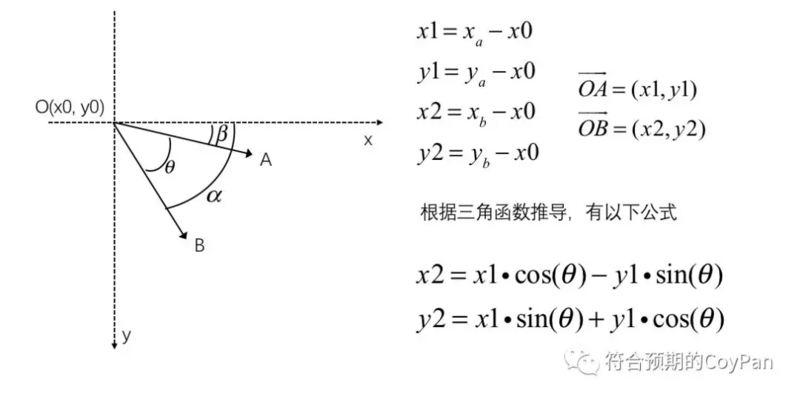``````/**
*
* 根据旋转起点、旋转中心和旋转角度计算旋转终点的坐标
*
* @param {Array} startPoint  起点坐标
* @param {Array} centerPoint  旋转点坐标
* @param {number} angle 旋转角度
*
* @return {Array} 旋转终点的坐标
*/

function getEndPointByRotate(startPoint, centerPoint, angle) {
const [centerX, centerY] = centerPoint;
const [x1, y1] = [startPoint - centerX, startPoint - centerY];
const x2 = x1 * Math.cos(angle) - y1 * Math.sin(angle);
const y2 = x1 * Math.sin(angle) + y1 * Math.cos(angle);
return [x2 + centerX, y2 + centerY];
}``````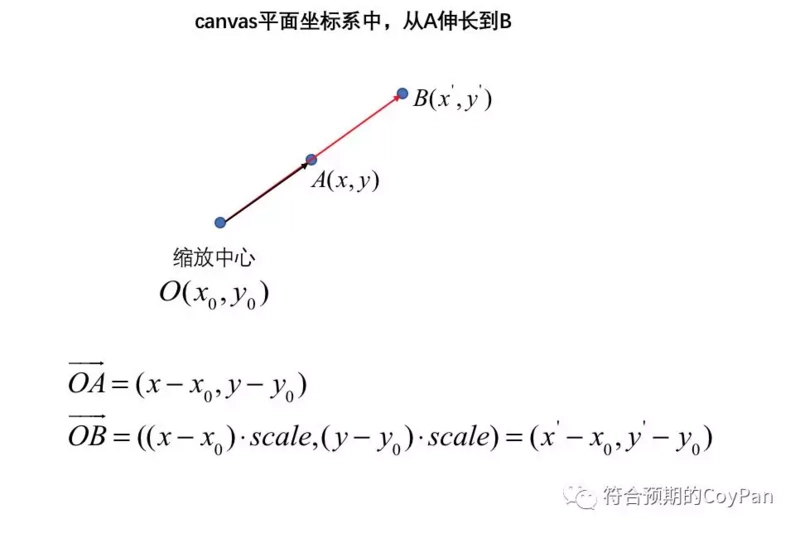6

2条回复

/

wx62579090b7e50

2022-4-14 11:11:16帖子
视频
声望
粉丝
社区精华内容